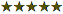Price per Set: \$?.??/Set , 1 Set = 4 Pads Of 50 Sheets

 Quantity: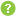0 = \$0.00 1 = \$28.95 2 = \$38.95 3 = \$48.95 4 = \$58.95 5 = \$68.95 6 = \$78.95 7 = \$88.95 8 = \$98.95 9 = \$108.95 10 = \$118.95 11 = \$128.95 12 = \$138.95 13 = \$148.95 14 = \$158.95 15 = \$168.95 16 = \$178.95 17 = \$188.95 18 = \$198.95 19 = \$208.95 20 = \$218.95 21 = \$228.95 22 = \$238.95 23 = \$248.95 24 = \$258.95 +MORE Printing Process:Digital Self-Stick Pads
Item # 4009_16 Memos Flat/Matte Ink 4" x 3"

Description

## Details

Self - Stick Memo Pads - Design 16

Pad Size: 3 x 4 Inches

Comes with 4 pads of 50 sheets each

Paper Color: White, Ivory, Yellow, Blue, Pink, Gray, Mint and Lavender

Reviews

Be the first to review this product

### You're reviewing:

 1 star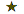2 stars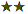3 stars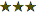4 stars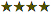5 stars